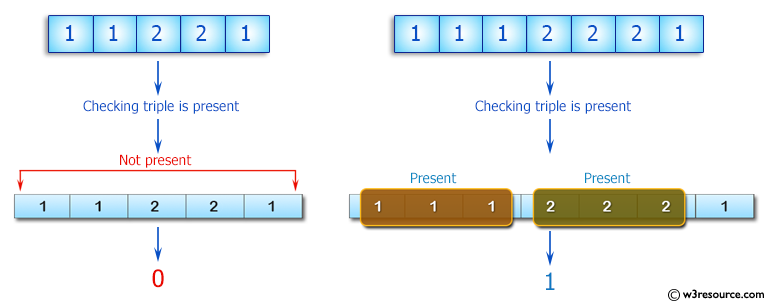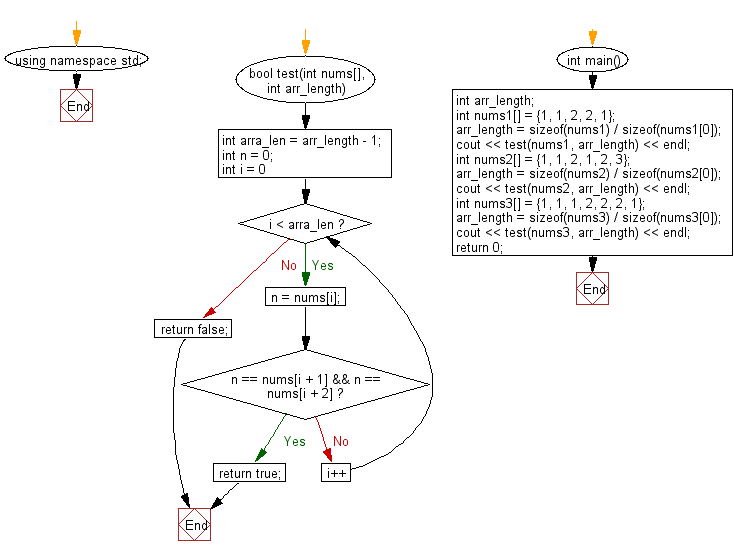﻿ C++ : Check if a triple is presents in an array or not# C++ Exercises: Check if a triple is presents in an array of integers or not

## C++ Basic Algorithm: Exercise-36 with Solution

Write a C++ program to check if a triple is presents in an array of integers or not. If a value appears three times in a row in an array it is called a triple.

Sample Solution:

C++ Code :

``````#include <iostream>

using namespace std;

bool test(int nums[], int arr_length)
{
int arra_len = arr_length - 1;
int n = 0;
for (int i = 0; i < arra_len; i++)
{
n = nums[i];
if (n == nums[i + 1] && n == nums[i + 2]) return true;
}
return false;
}

int main()
{
int arr_length;
int nums1[] = {1, 1, 2, 2, 1};
arr_length = sizeof(nums1) / sizeof(nums1);
cout << test(nums1, arr_length) << endl;
int nums2[] = {1, 1, 2, 1, 2, 3};
arr_length = sizeof(nums2) / sizeof(nums2);
cout << test(nums2, arr_length) << endl;
int nums3[] = {1, 1, 1, 2, 2, 2, 1};
arr_length = sizeof(nums3) / sizeof(nums3);
cout << test(nums3, arr_length) << endl;
return 0;
}
``````

Sample Output:

```0
0
1
```

Pictorial Presentation:Flowchart:C++ Code Editor: